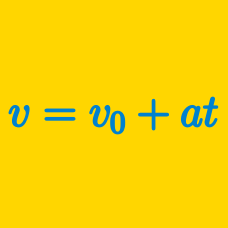Classical Mechanics

Position-time graph - constant velocity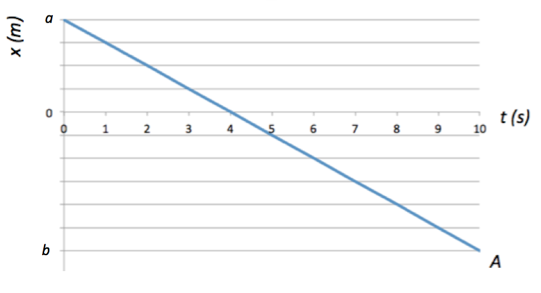The position of particle $A$ moving along the $x$-axis is given by the above diagram, where the vertical scalings are $a = 32 \text{ m}$ and $b= -48 \text{ m}.$ Assuming that particle $A$ keeps moving in the same manner, find the position of particle $A$ at $t = 11 \text{ s}.$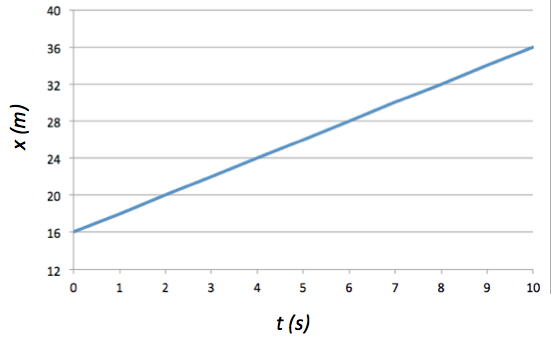The above diagram is the position versus time graph of a particle which is moving along the $x$-axis. Find the velocity of the particle at $t= 7 \text{ s}.$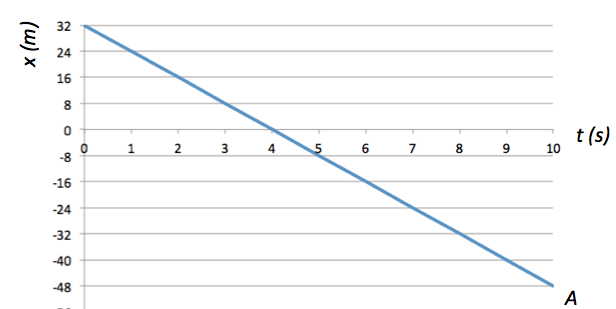The position of particle $A$ moving along the $x$-axis is given by the above diagram. Assuming that particle $A$ keeps moving in the same manner, find the position of particle $A$ at $t = 34 \text{ s}.$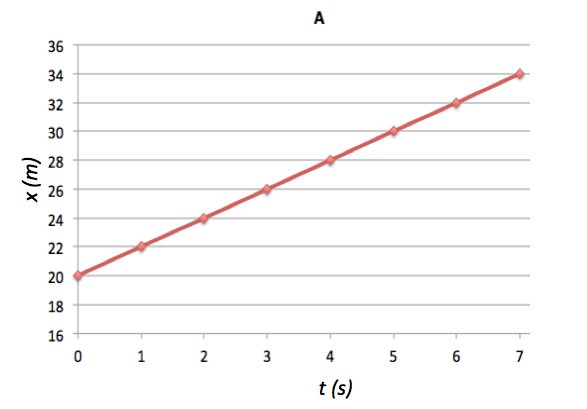Cars $A$ and $B$ move in the same direction in adjacent lanes. The position $x_A$ of car $A$ from $t = 0 \text{ s}$ to $t = 7.0 \text{ s}$ is given in the above graph, Car $B$ moves at a constant velocity of $8 \text{ m/s}$ and its position at $t =0 \text{ s}$ is $x_B = 0.$ Find the time when car $B$ gets ahead of car $A.$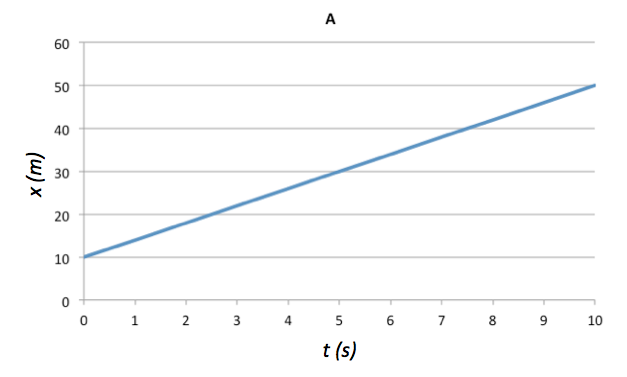The position of particle $A$ moving along the $x$-axis is given by the above diagram, from $t = 0 \text{ s}$ to $t = 10 \text{ s}.$ Which of the following correctly manifests the position of particle $A$ in terms of $t?$

×